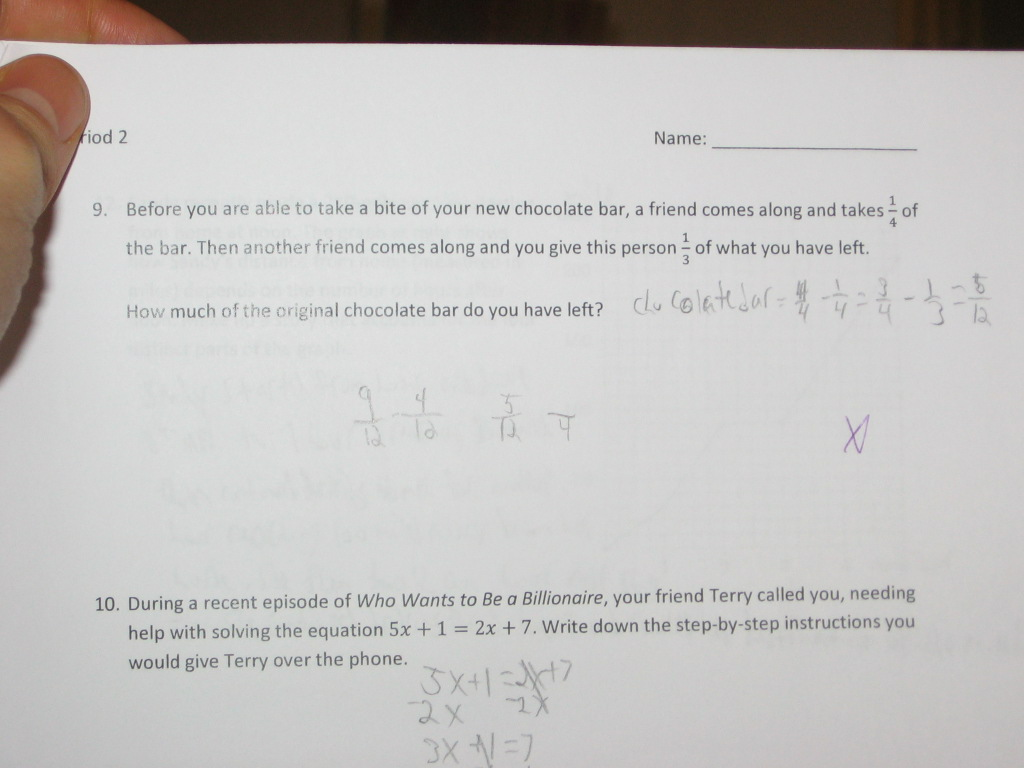Categories

# Chocolate Bars and Bad FriendsWhat’s the fastest way to help this 9th grade student?

## 5 replies on “Chocolate Bars and Bad Friends”

I’d bet that a visual model would help this student a tonne. Also, this may be a problem with reading, not necessarily math. They may have just missed the 1/3 “of what you have left” or not understood how this is important.

I agree. This is a problem that my ELL students have difficulty with. I have them write “now i have$\dfrac {3} {4}$ (or 0.75) remaining” and “I keep$\dfrac {2} {3}$ of that amount.” I got rid of “left” and put in “remains” or “remaining” after I heard about the left angle triangle, and realized that those dual-use words are a nightmare for my kids.

Units!

I would do some messing around with things like pounds and ounces of chocolate chips, and then cases and individual bars of chocolate, and then point out that you need to be especially careful with units in situations where you have percents or sometimes fractions. What unit is it a fraction of?

Definitely visual models.

What makes this confusing for students is that the first step of 4/4-1/4 does give the correct answer of 3/4. The student doesn’t understand that it works because they are subtracting 1/4 of 4/4, and that next they need to subtract 1/3 of 3/4. Simple subtraction works with whole numbers (You have 15 candies, your friend takes 3, and then another friend takes 5 more). This student needs to develop the idea that taking away fractional parts is more complicated.Laura Adlersays:

The key is in the phrase “of what you have left”. The one-third is being taken from a different whole (3/4 of the original whole)–but the amount remaining is defined by the original whole. In a subtraction problem, the size of the 1/3 would be in relation to the whole which is what this student did.

In my opinion the best way to model this is using bar models – I have an example but I’m not sure how to post it here. I’ll try to describe what this looks like: First draw a bar partitioned into 4 equal-size parts. Second, one part is given to the first friend. What’s left is a bar with three equal sized pieces. Third, friend two takes one of these three parts and you are left with two equal size parts. In order to describe the quantity left, this remaining part is placed on top of the original bar and it’s obvious that it equals one-half of the original bar.

Bar models (also known as Singapore Bar Models) really help kids see what is going on in these types of problems. The next step is to make a similar model that shows what the student did in his/her solution and compare the solutions. It’s not a guarantee that the student will connect this model to the symbolic process right away but at least students can conceptually visualize and think through such problems.

If this is not clear and you would like to see an example, please e-mail me.